## Identification and Analysis of Binary Star Systems using Probability Theory

E. Grocheva and A. Kiselev
Photographic Astrometry Department, Pulkovo Observatory, S.Petersburg, 196140, Russia, Email: gl@spb.iomail.lek.ru

### Abstract:

Accurate identification of physical binary star systems has become of increasing interest in recent years. The frequency of occurrence of binary and multiple star systems remains uncertain at the present time, and is a stumbling block to our understanding of the formation of stars, stellar systems, and planetary systems. Identification of physical binary star systems, with an estimated reliability of such identifications based on the theory of probability, is also important for current and future efforts to find binary star systems that are candidates for observations with the aim of determining their orbits and component masses.

We shall present methods which allow the identification of physical binary stars in a probabilistic sense. The application of probability theory provides a more complete picture of the frequency of stellar binarity than simple methods based only on proximity or proper motion. We will also present preliminary results from the application of these methods to Pulkovo's observation program of binary star systems, and outline how such methods might be applied to present and future high precision astrometric catalogues.

### 1. Introduction

It is usually difficult to identify physical binary star systems without long-term observations. A simple comparison of proper motions does not provide certain verification of the connection between the components. In many cases we do not know the distances to stars or stellar space velocities. Therefore two stars seen in the same direction and having similar proper motions may have different distances and space velocities. Estimating the component masses is interesting from the point of view of searching for hidden masses. It is possible to make this identification on the basis of probability theory. We propose to estimate the probability P of the random disposition of two or more stars, having similar proper motions, on small angular distance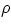. True physical systems will have the lowest such probabilities.

### 2. Estimation of Probability.

We propose to use the real distribution of proper motions for estimation of P. Let the probability of finding the primary component in the area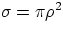equal 1 as a probability of a reliable event. The probability P of finding n components in a small area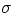may be represented as: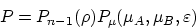(1)

where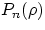- probability of finding n stars in small area limited with angular distancefor the case of a random distribution, (Deutsch,1962):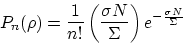(2)

n - is multiplicity of a star system;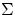- is area where N stars are randomly disposed;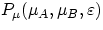- probability of the proximity of proper motions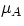and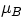;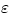- error of determination of proper motions.

Let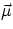be a random vector,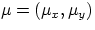, where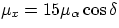,. Iftakes on a value from the cell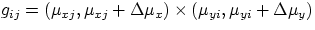, then we say that random vectortakes the value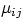. Hence, vector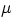may take the following values: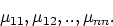Let the probabilities thattakes one or another value be equal correspondingly to

p11,p12,..,pnn.

and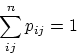i.e., the density of probability of the random vector is known. In the case of statistical estimation of probabilities the quantities pij are the relative frequencies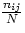, thenis equal to: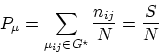(3)

where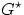is the Borel's set, where the tips of vectors are situated at: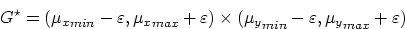(4)

Now we will describe a procedure for the solution of this problem without a concrete realisation.

• Using any astrometric catalogue with stellar positions and proper motions we obtain the differential law of distribution (density of probability) of proper motions. We construct the matrix M whose elements will be nij (3). Let us consider the stars whose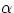and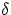satisfy certain specified conditions, for example,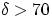. Properly speaking, selection conditions can be very different, but for the present we solve this problem from an astrometric point of view only. For every star we calculate the position of corresponding element in matrix M. We add 1 to the element thus found. The elements of resulting matrix will yield the probability density of proper motion distribution.
• Let us consider the binary star whose components have proper motions,and angular spacing. The required probability is equal to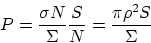according to (1) -(3).
• We choose assumed binaries from some catalogue. The sample must be restricted to pairs whoseare limited by some quantity. For example it is possible to use the Aitken's criterion(Aitken,1932). Then we determine the probability of random distribution for every pair. The sample must include few known optical and physical pairs to obtain a definite criterion for the identification.

### 3. Results of Analysis of Stars from Pulkovo's Observation Program.

The distribution of proper motions was derived from the PPM catalogue for stars of the North-polar area. The parameters of the distributions are in Table 1. The predominance of negative motions, especially of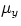, is readily observable. This is due to the location of the North-polar area relative to the Solar apex. We chose 76 double stars from Pulkovo's observation program and calculated the probabilities of a random distribution for these pairs. There are 8 physical and 12 optical systems among them (Grocheva,1996 & Catalogue of relative positions and motions of 200 visual double stars,1988). The proper motions of these pairs was obtained by using the catalogue Carte du Ciel'' and modern observations with the 26''refractor. The precision ofis 0''.005 /yr.

 Mean Variance max min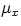-0''.0006 0.047 1''.545 -2''.97-0''.0059 0.041 0''.818 -1''.74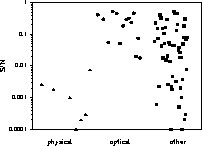Analysing the resulting probabilities we conclude that only the probability of random proximity of proper motions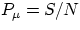can be used to identify true physical binaries. Multiplication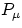by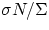corrupts the probability pattern. Figure 1 shows probabilitieson a logarithmic scale (we numbered the pairs of this sample from 1 to 76 and used these numbers as x-coordinates). We see that probabilities for physical pairs are less than 0.01, whilst those for optical ones are large. Hence, the quantitymay be used to identify physical binaries and the limit of probability of proper motions proximity S/N is 0.01 for physical pairs. It turned out that only 27 physical binaries were among the sample of 76 double stars.

### 4. Conclusion.

The technique presented identifies physical binaries. This method has a simple algorithm and can be used for the automatic treatment of large stellar catalogues. We are pleased to also note that this method requires only minimal data such as positions and proper motions. This method was used to correct the Pulkovo's program of binary star observations.

### References:

Deutsch, A. N., 1962, The visual double stars''. in The course of astrophysics and stellar astronomy, p.60, Moscow, (in Russian).

Catalogue of relative positions and motions of 200 visual double stars.,1988, Saint-Petersburg, (in Russian).

Grocheva, E. A., 1996, Physical and optical double stars...'', Workshop The Visual Double Stars.., Spain.

Aitken, R. G., 1932, New General Catalogue of Double Stars, Edinburgh

© Copyright 1998 Astronomical Society of the Pacific, 390 Ashton Avenue, San Francisco, California 94112, USA

Next: Modelling Spectro-photometric Characteristics of Eclipsing Binaries
Up: Computational Astrophysics
Previous: Modelling Spectro-photometric Characteristics of Nonradially Pulsating Stars